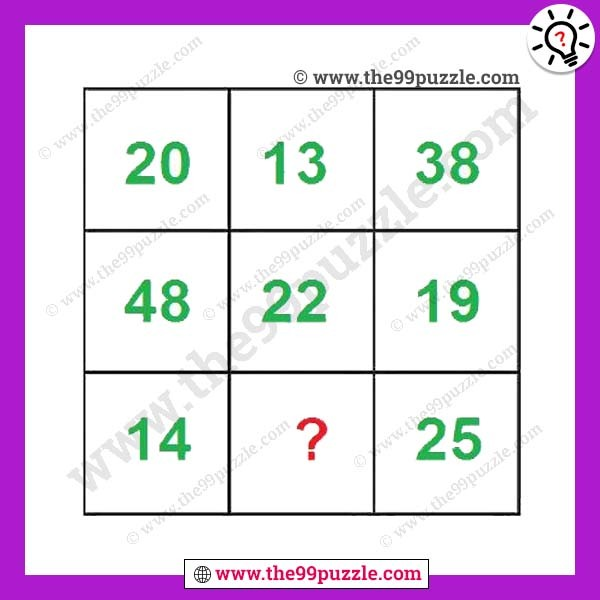# Interesting Maths Equation Puzzle With Answer – Puzz107

This interesting maths equation puzzle can increase your solving skills. Maths Logic Brain Teaser will challenge your mind. Observe the pattern in the given number equation and then find the value of the missing number which replaces the question mark. Can you solve this Maths Brain Teaser?###### Explanation:

In this math puzzle, the logic is each column all numbers sum is 82

1st column = 20+48+14=82

2nd column = 13+22+47=82

3rd column = 38+19+25=82

The math problem is important ways such as relaxation and enjoyment. We create the Math Puzzle in a different logic. Many Puzzle lovers enjoy the Math Puzzle Logic which is to improve your memory. Math Puzzles can improve your logical thinking and problem-solving skills.

Here you will find many , and many more puzzles. We are creating various fun and interesting maths equation puzzle for adults and kids. Always think logically to solve easily these types of puzzles. On this website, you will play very fun games & quizzes that can improve your visual and logical thinking. Regularly we are updated with new puzzles & quizzes on this site. Check out daily to get new puzzles, riddles & quizzes.

1.